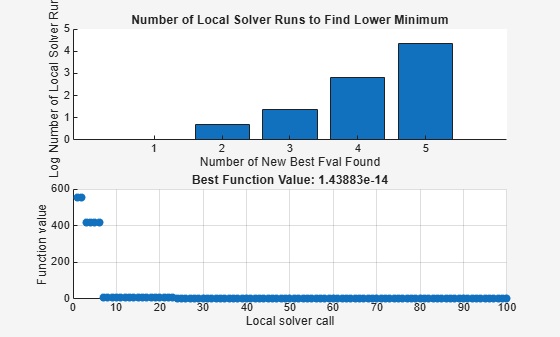Main Content

## Plot Functions for GlobalSearch and MultiStart

### What Are Plot Functions?

The `PlotFcn` field of `options` specifies one or more functions that an optimization function calls at each iteration. Plot functions plot various measures of progress while the algorithm executes. Pass a function handle or cell array of function handles. The structure of a plot function is the same as the structure of an output function. For more information on this structure, see OutputFcn.

Plot functions are specialized output functions (see Output Functions for GlobalSearch and MultiStart). There are two predefined plot functions:

• `@gsplotbestf` plots the best objective function value.

• `@gsplotfunccount` plots the number of function evaluations.

Plot function windows have and buttons. By default, all plots appear in one window.

To use global plot functions:

• Write plot functions using the syntax described in OutputFcn.

• Set the `PlotFcn` property of your `GlobalSearch` or `MultiStart` object to the function handle of your plot function. You can use multiple plot functions by setting the `PlotFcn` property to a cell array of function handles.

#### Details of Built-In Plot Functions

The built-in plot functions have characteristics that can surprise you.

• `@gsplotbestf` can have plots that are not strictly decreasing. This is because early values can result from local solver runs with negative exit flags (such as infeasible solutions). A subsequent local solution with positive exit flag is better even if its function value is higher. Once a local solver returns a value with a positive exit flag, the plot is monotone decreasing.

• `@gsplotfunccount` might not plot the total number of function evaluations. This is because `GlobalSearch` can continue to perform function evaluations after it calls the plot function for the last time. For more information, see GlobalSearch Algorithm.

### `MultiStart` Plot Function

This example plots the number of local solver runs it takes to obtain a better local minimum for `MultiStart`. The example also uses a built-in plot function to show the current best function value.

The example problem is the same as in Find Global or Multiple Local Minima, with additional bounds.

The example uses persistent variables to store previous best values. The plot function examines the best function value after each local solver run, available in the `bestfval` field of the `optimValues` structure. If the value is not lower than the previous best, the plot function adds 1 to the number of consecutive calls with no improvement and draws a bar chart. If the value is lower than the previous best, the plot function starts a new bar in the chart with value 1. Before plotting, the plot function takes a logarithm of the number of consecutive calls. The logarithm helps keep the plot legible, since some values can be much larger than others.

To store local results using nested functions instead of persistent variables, see Example of a Nested Output Function.

Plot Function Example

This example minimizes the `sawtoothxy` helper function, which is listed at the end of this example. In general, save your objective function in a file on your MATLAB® path.

The `NumberToNextBest` custom plot function is attached to this example. In general, save your plot function in a file on your MATLAB path. Here is a listing.

`type NumberToNextBest`
```function stop = NumberToNextBest(optimValues, state) persistent bestfv bestcounter stop = false; switch state case 'init' % Initialize variable to record best function value. bestfv = []; % Initialize counter to record number of % local solver runs to find next best minimum. bestcounter = 1; % Create the histogram. bar(log(bestcounter),'tag','NumberToNextBest'); xlabel('Number of New Best Fval Found'); ylabel('Log Number of Local Solver Runs'); title('Number of Local Solver Runs to Find Lower Minimum') case 'iter' % Find the axes containing the histogram. NumToNext = ... findobj(get(gca,'Children'),'Tag','NumberToNextBest'); % Update the counter that records number of local % solver runs to find next best minimum. if ~isequal(optimValues.bestfval, bestfv) bestfv = optimValues.bestfval; bestcounter = [bestcounter 1]; else bestcounter(end) = bestcounter(end) + 1; end % Update the histogram. set(NumToNext,'Ydata',log(bestcounter)) end ```

Create the problem structure and global solver object. Set lower bounds of `[-3e3,-4e3]`, upper bounds of `[4e3,3e3]` and set the global solver to use the `NumberToNextBest` custom plot function and the `gsplotbestf` built-in plot function.

```problem = createOptimProblem('fmincon',... 'objective',@(x)sawtoothxy(x(1),x(2)),... 'x0',[100,-50],'lb',[-3e3 -4e3],... 'ub',[4e3,3e3],'options',... optimoptions(@fmincon,'Algorithm','sqp')); ms = MultiStart('PlotFcn',{@NumberToNextBest,@gsplotbestf});```

Run the global solver for 100 local solver runs.

```rng(2); % For reproducibility [x,fv] = run(ms,problem,100);``````MultiStart completed some of the runs from the start points. 34 out of 100 local solver runs converged with a positive local solver exit flag. ```

Helper Functions

This code creates the `sawtoothxy` helper function.

```function f = sawtoothxy(x,y) [t,r] = cart2pol(x,y); % change to polar coordinates h = cos(2*t - 1/2)/2 + cos(t) + 2; g = (sin(r) - sin(2*r)/2 + sin(3*r)/3 - sin(4*r)/4 + 4) ... .*r.^2./(r+1); f = g.*h; end```

### No Parallel Plot Functions

While `MultiStart` can run in parallel, it does not support global output functions and plot functions in parallel. Furthermore, while local output functions and plot functions run on workers when `MultiStart` runs in parallel, the effect differs from running serially. Local output and plot functions do not create a display when running on workers. You do not see any other effects of output and plot functions until the worker passes its results to the client (the originator of the `MultiStart` parallel jobs).

For information on running `MultiStart` in parallel, see Parallel Computing.

## SupportGet trial now